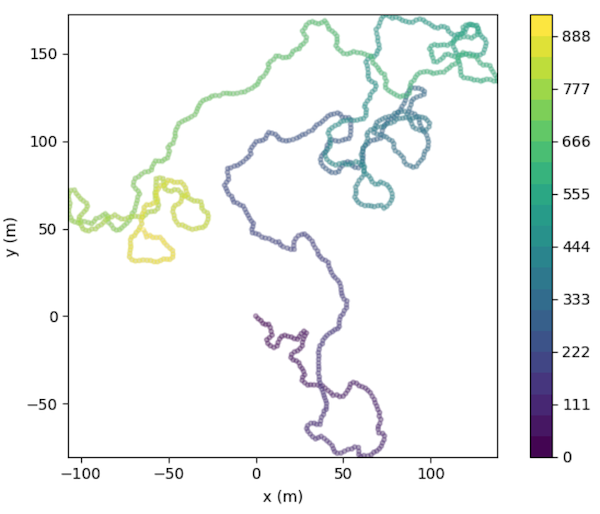# Generate Random Walk¶

Random walks can be generated using `generate()`.

```In : import traja

# Generate random walk
In : df = traja.generate(1000)
```
traja.trajectory.generate(n: int = 1000, random: bool = True, step_length: int = 2, angular_error_sd: float = 0.5, angular_error_dist: = None, linear_error_sd: float = 0.2, linear_error_dist: = None, fps: float = 50, spatial_units: str = 'm', seed: = None, convex_hull: bool = False, **kwargs)[source]

Generates a trajectory.

If `random` is `True`, the trajectory will be a correlated random walk/idiothetic directed walk (Kareiva & Shigesada, 1983), corresponding to an animal navigating without a compass (Cheung, Zhang, Stricker, & Srinivasan, 2008). If `random` is `False`, it will be(np.ndarray) a directed walk/allothetic directed walk/oriented path, corresponding to an animal navigating with a compass (Cheung, Zhang, Stricker, & Srinivasan, 2007, 2008).

By default, for both random and directed walks, errors are normally distributed, unbiased, and independent of each other, so are simple directed walks in the terminology of Cheung, Zhang, Stricker, & Srinivasan, (2008). This behaviour may be modified by specifying alternative values for the `angular_error_dist` and/or `linear_error_dist` parameters.

The initial angle (for a random walk) or the intended direction (for a directed walk) is `0` radians. The starting position is `(0, 0)`.

Parameters
• n (int) – (Default value = 1000)

• random (bool) – (Default value = True)

• step_length – (Default value = 2)

• angular_error_sd (float) – (Default value = 0.5)

• angular_error_dist (Callable) – (Default value = None)

• linear_error_sd (float) – (Default value = 0.2)

• linear_error_dist (Callable) – (Default value = None)

• fps (float) – (Default value = 50)

• convex_hull (bool) – (Default value = False)

• spatial_units – (Default value = ‘m’)

Returns

Trajectory

Return type

trj (`traja.frame.TrajaDataFrame`)

Note

Based on Jim McLean’s trajr, ported to Python.

Reference: McLean, D. J., & Skowron Volponi, M. A. (2018). trajr: An R package for characterisation of animal trajectories. Ethology, 124(6), 440-448. https://doi.org/10.1111/eth.12739.